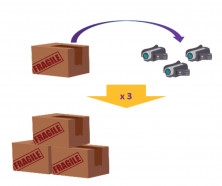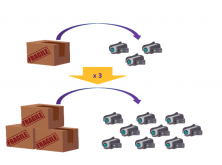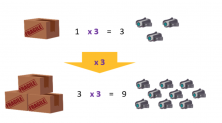Smartick is an advanced online program that teaches kids math and coding in only 15 min. a day
Try it for free!

Apr13

# Learn the Basic Concepts of the Rule of 3

In this post, we’re going to take a look at when we can use the rule of 3 as a tool to solve a problem.

The rule of 3 is one of the most applicable and most useful mathematical phenomena, above all due to its combination of simplicity and efficiency.

We should take into account that the rule of 3 is based on the type of relationship that exists between two quantities. For example:

####In this figure, we can see that a numerical relation exists between the box and the video cameras it contains. The relation being “1 box contains 3 video cameras.”

Knowing this, we can now solve the following problem.How many video cameras are there in three boxes?

We can only solve this problem because we know the number of video cameras in each box, therefore we apply this relation to the three boxes.

“If there are 3 cameras in one box, three boxes will have the same contents as one box multiplied by 3.”This method of reasoning is what we call in mathematics “the rule of 3.”All we have done is use the information from the first situation to solve the second.Now you know what the rule of 3 is. If you want to see more examples and practice exercises, sign up for a free trial at Smartick!

Learn More:

Latest posts by Smartick (see all)